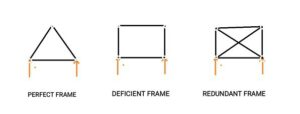# What is difference between deficient frame and redundant frame?

Before knowing difference between both frame, you should visualize a perfect frame.

A perfect frame is the frame which is composed of such members, which are just sufficient to keep the frame in equilibrium, when the frame is supporting an external load. Triangle is the simplest perfect frame.Here,

AB, BC and AC are members and A, B and C are joints.

If we add a set of two members and a joint in a perfect frame, we get another perfect frame.For a perfect frame,

n=2j3n=2j−3

Where, nn= number of members and jj= number of joints.

Now, if in a frame, number of members and number of joints are not given by n=2j3n=2j−3 then it is called imperfect frame.

Deficient frame and redundant frame are imperfect frame.

• If n<2j3n<2j−3 then it is known as deficient frame.
• If n>2j3n>2j−3 then it is known as redundant frame.Open